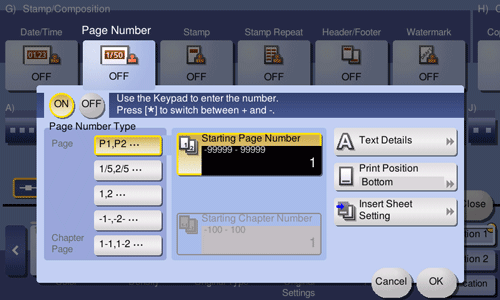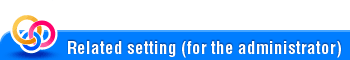HomeDescriptions of Functions / Utility KeysPage Number

# Page Number

##### To display:
• [Classic Style]
• [Copy]
• [Application]
• [Stamp/Composition]
• [Page Number]

Adds page and chapter numbers on printed sheets. Page and chapter numbers are printed on all pages.Setting

Description

[ON]/[OFF]

Select [ON] to set Page Number.

[Page Number Type]

Select the format of the page number.

[Starting Page Number]

Specify the starting page number to be printed on the first page.

For example, to print page numbers from the third page of the file, enter "-1". The first page of the file is counted as "-1", the second page as "0", and the third page is counted as "1". In [Starting Page Number], a page number is printed on pages 1 and later. If "2" is entered, page numbers are printed from the first page of the file as "2, 3", etc. in order. "-" (minus sign) switches "+" and "-" each time you tap [*].

[Starting Chapter Number]

Specify the starting chapter number if a file is divided into chapters, and you want to print chapter numbers from the second chapter. You can specify starting chapter numbers when you have selected [Chapter Page] in [Page Number Type].

For example, to print chapter numbers from the third chapter, enter "-1". The first chapter of the file is counted as "-1", the second chapter as "0", and the third chapter is counted as "1". In [Starting Chapter Number], a chapter number is printed on the chapters that are count as 1 and later. If "2" is entered, a number is printed from the first page of the first chapter of the file as "2-1, 2-2". "-" (minus sign) switches "+" and "-" each time you tap [*].

[Text Details]

If necessary, select the print size, font, and other conditions.

[Print Position]

If necessary, select the print position of the text. You can more finely adjust the print position in [Adjust Position].

[Insert Sheet Setting]

When you insert sheets using the Cover Sheet function of Insert Sheet function, specify whether or not to print a page number on the inserted sheet.

• [Cover Sheet]: Select whether to print a page number on a cover sheet. A cover page is counted as the number of pages even if [Do not print] is selected.

• [Insert Sheet (Copy)]: Prints - or does not print - a page number on the inserted copy paper. The inserted pages are counted as the number of pages even if [Do Not Print Number] is selected. If [Skip] is selected, the page number is not printed, and the inserted page is not counted as the number of pages.

• [Insert (Blank)]: Select whether to count a blank page as the number of pages when inserting blank paper. The inserted pages are counted as the number of pages if [Do Not Print Number] is selected. If the [Skip] is selected, the inserted pages are not counted as the number of pages.

#####• [Blank Page Print Settings] (Here)